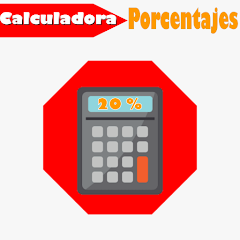100+Everyone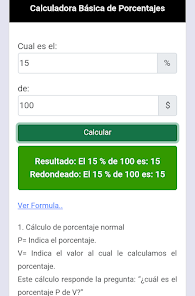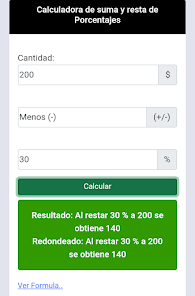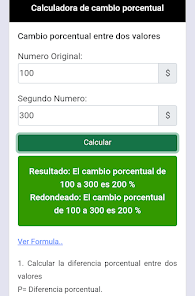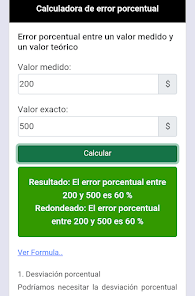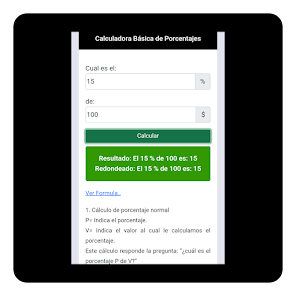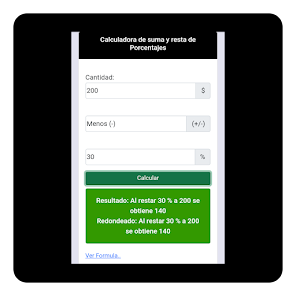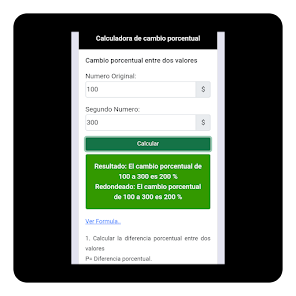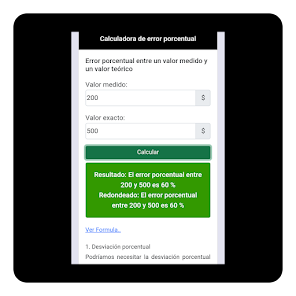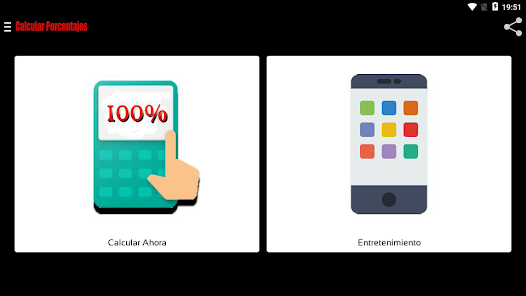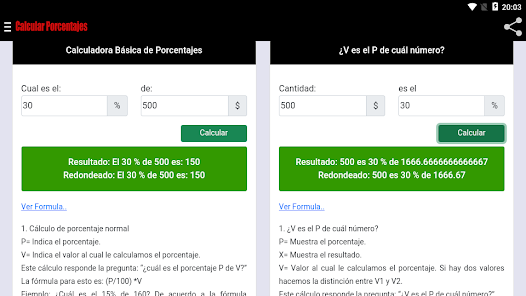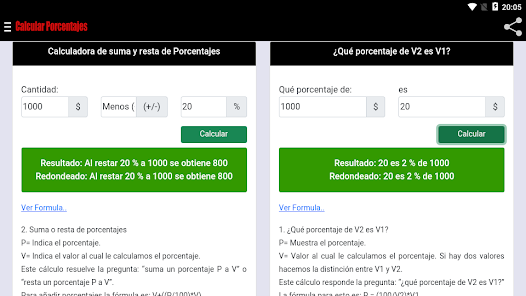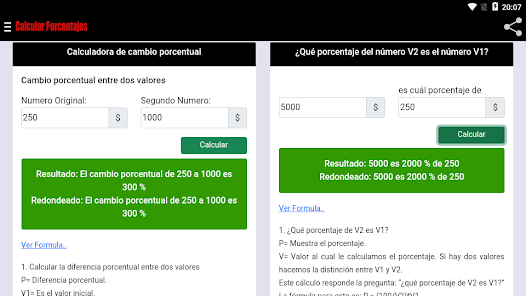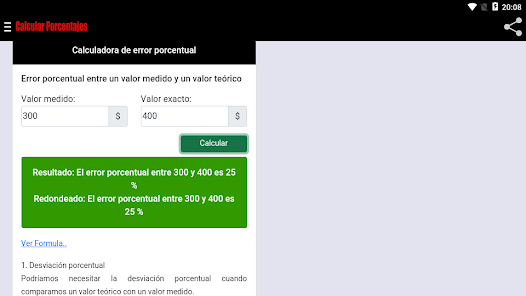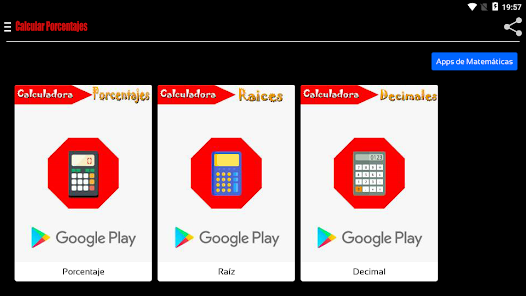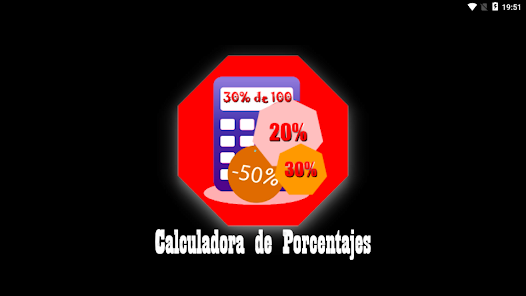Discover the "Percentage Calculator" application, which will allow you to calculate a percentage and know the formulas applied correctly for free with just a few clicks. 👍

To be used during sales, calculations, sales, on the go or at work.

This percentage calculator allows you to calculate the:
* simple or basic percentage calculator ( 5 percent of 40 is 20)
* percent decrease (5 percent decrease from 40 is 38)
* percent increase (5 percent increase from 40 is 42)
* percentage change between two values
* percentage error between a measured value and a theoretical value
* V is the P of which number?
* What percentage of V2 is V1?
* What percentage of the number V2 is the number V1?

Other calculations and functions:
- Basic Percentage Calculator
- Calculator of addition and subtraction of Percentages
- Percent Change Calculator
- Percent error calculator
- Calculator of V is the P of which number?
- Calculator of What percentage of V2 is V1?
- Calculator of What percentage of the number V2 is the number V1?
- Discount calculator.
- Increase Percentage Calculator
- Decrease Percentage Calculator
- Calculator of percentage of an amount
- Calculator for converting percentages into decimals or fractions
- Definition and Concept of Percentage
- How to calculate the percentage?
- Formula for converting percentages into decimals or fractions
- Discount Calculation Formula
- Includes all the Formulas of the calculations made

- Gross income tax
- Discount voucher for a certain amount
- Discount coupon of a certain percentage
- Calculation of tips
- Interest on a bond
- Percentage increase in a savings account
- Percentage decrease after a price reduction
- Difference between measured values ​​and theoretical values
- Deviation after rounding

Use:
The application offers a simple control panel allowing you to choose the percentage calculation you want by sliding down you will find the other calculators and the definitions, formulas, uses etc. The results are obtained with complete and rounded decimals.

The percentage calculation is performed as soon as you click "Calculate" after entering the following basic information:
- Initial Value (Amount \$100)
- Percentage (10%)

✅ Tip:
- You should only add the necessary data to calculate and solve the problem, no special characters or symbols are required, just add the amounts of your operation.

-------------------------------------------------- -------------------------------------------------- ----------------------

How to calculate a percentage (or how to get a percentage) according to the 3 calculation methods:

- Percent Increase Calculator:
There are several calculation methods, this is one of them:
Marc has a salary of \$1000 USD and wants to know his new salary with a 12.5% ​​increase. So we take 12.5/100 or 0.125 and multiply it by the salary 1000x0.125. We discovered an increase of \$125 USD, so your new salary will be \$1125 USD.

- Decrease Percentage Calculator:
Kevin spends \$40 USD a week on gas, and he wants to reduce his expenses by 15%. So, based on 100%, we subtract the 15% you want to save, leaving you with 85%, divide by 100 (85/100 = 0.85), and multiply by \$40 USD.
This gives us 40 x 0.85 = \$34 USD (So you would save \$6 USD).

-------------------------------------------------- --------------------------------------------

Note: An internet connection, 3G/4G or WiFi is required.

ℹ️ SUPPORT
For faster and more effective communication, if you experience any problems or if you cannot find what you are looking for, send us an email to appmovil.tecnologia@gmail.com.

If you liked the application, we would appreciate a positive assessment. 👍 Thank you very much!
Updated on
Sep 15, 2022

## Data safety

Safety starts with understanding how developers collect and share your data. Data privacy and security practices may vary based on your use, region, and age. The developer provided this information and may update it over time.No data shared with third partiesNo data collected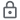Data is encrypted in transit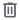You can request that data be deleted

## What's new

Esta calculadora de porcentaje le permite calcular el:
* calculadora de porcentaje simple o básico ( 5 porciento de 40 es 20)
* porcentaje de disminución (5 porciento de disminución de 40 es 38)
* porcentaje de incremento (5 porciento de incremento de 40 es 42)
* cambio porcentual entre dos valores
* error porcentual entre un valor medido y un valor teórico
* ¿V es el P de cuál número?
* ¿Qué porcentaje de V2 es V1?
* ¿Qué porcentaje del número V2 es el número V1?
* calcular porcentaje gratis